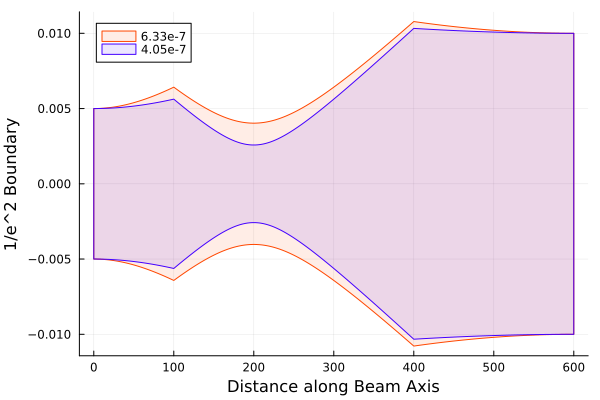# [ANN] JuliaPhysics/ABCDMatrixOptics.jl - Ray Transfer Matrix Analysis

Hi all,

ABCDMatrixOptics.jl is small package which implements linear tracing of simple optical systems based on the Ray transfer matrix analysis.
The convention of the optical elements is identical to those on Wikipedia. So far we allow most standard optical elements and geometric and Gaussian beams.
It evolved from the ABCDBeamTrace.jl and should match its features.

## Simple Example

``````using ABCDMatrixOptics

# create needed optical elements
f1 = FreeSpace(200)
l1 = ThinLens(200.0)
f12 = FreeSpace(200 + 400)
l2 = ThinLens(400.0)
f2 = FreeSpace(400)

# simple, single ray
beam = GeometricBeam(w=10.0, k=0.1)

# create optical system
# it's built from left to right.
M = [f1, l1, f12, l2, f2]

# apply the system to the beam
# The matrices are evaluates as f2 * l2 * f12 * l1 * f1 * beam
# * is syntactic sugar for propagate
beam_p = M * beam

# GaussianBeam
red_beam = GaussianBeam(w0=5e-3)
blue_beam = GaussianBeam(w0=5e-3, λ=405e-9)

using Plots

plot(M, red_beam)
plot!(M, blue_beam)
``````This package evolved as a community effort so feel free to submit PRsAlso I strongly believe, we should add more Physics related packages to JuliaPhysics to increase visibility!

Best,

Felix

8 Likes